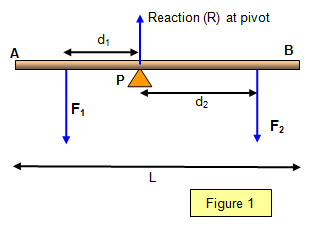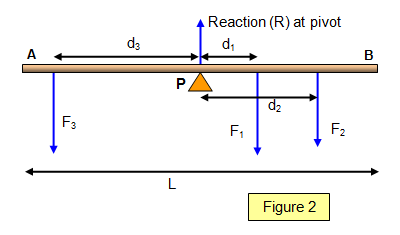# Equilibrium

A body is truly in equilibrium when it has no tendency to turn or move. This means no translation and no rotation.

When a body is in equilibrium:

The sum of the anticlockwise moments about any point is equal and opposite to the sum of the clockwise moments about that point.

and also:The resultant force in any direction is zero.

It is easy to show this using the light loaded beam of length L shown in Figure 1. Notice that F1 will turn the beam anticlockwise about the pivot (P) while F2 will turn it clockwise.

Using the principle of moments and taking moments about the pivot:

Clockwise moments = Anticlockwise moments      F2d2 = F1d1

Balancing the vertical forces:

Sum of the vertical forces is zero.      F1+ F2 – R = 0

Notice the minus sign since R acts in the opposite direction from F1 and F2.

Example problem
F1 = 20 N, d2 = 15 cm, d2 = 50 cm
Find the value of F2 and the reaction at the pivot such that the beam is in equilibrium.

(a) Take moments about the pivot:
Clockwise moments = 20x0.15 = F2x0.5 = Anticlockwise moments
Therefore: F2 = 6 N

(b) Taking the sum of the vertical forces to be zero: F1 + F2 - R = 0
R = 26 N

The same result would be obtained by taking moments about ANY point.

### Further example

A more complex example where there are three forces acting on the rod is shown below (Figure 2). The rod is pivoted about its centre.Using the principle of moments and taking moments about the pivot:

Clockwise moments = F1d1 + F2d2 = F3d3 = Anticlockwise moments

### Moments may be taken about any point

(a) If we take moments about end A :

Anticlockwise moments = LR/2 = [L/2 + d1] F1+ [L/2 + d2] F2 + [L/2 – d3] F3 = Clockwise moments

(b) If we take moments about end B :

Anticlockwise moments = [L/2 - d1] F1+ [L/2 - d2] F2 + [L/2 + d3] F3 = LR/2 = Clockwise moments

Combining equations (a) and (b) gives:

Clockwise moments = F1d1 + F2d2 = F3d3 = Anticlockwise moments

This is the identical result that we obtained by taking moments about the pivot.

Example problems
Let L = 100 cm, F1 = 20 N, F3 = 10 N, d1 = 10 cm, d2 = 70 cm, d3 = 45 cm
Find the values of F2 and R such that the beam is in equilibrium.